Home > CCA2 > Chapter 12 > Lesson 12.1.3 > Problem12-56

12-56.
1. Rewrite each sum below as a single expression. Homework Help ✎

1.+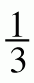2.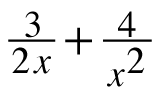3.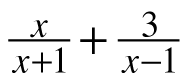4.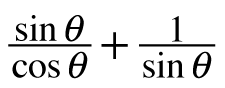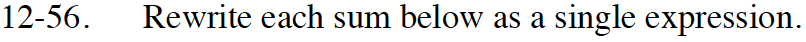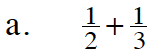Find a common denominator.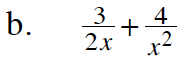What do you need to multiply each term by to have a common denominator? It's similar to part (a).

$\frac{3x + 8}{2x^2}$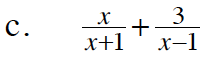Use the same method as part (b).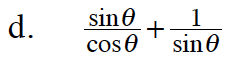$\frac{\sin^2\theta+\cos\theta}{\sin\theta\cos\theta}$